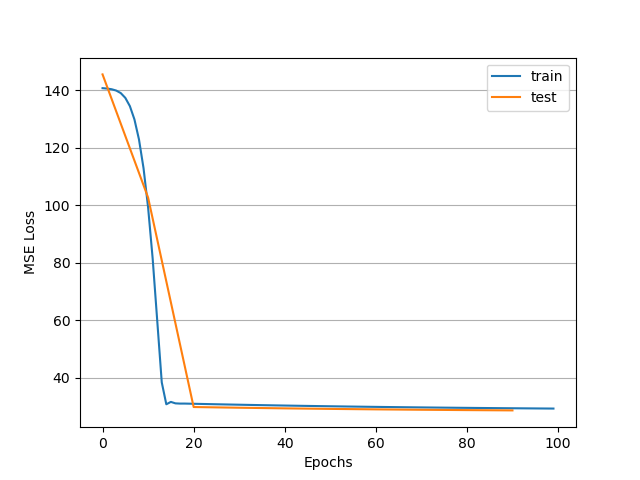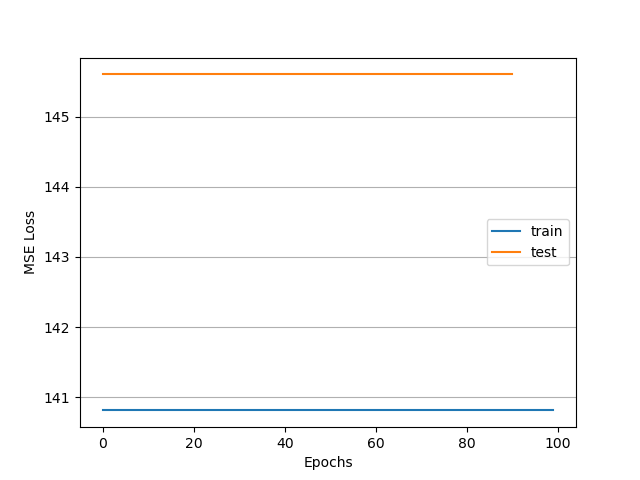# Training sometimes starts and remains with zero gradient values

I am observing the gradients associated with my neural network weights and sometimes they hold a value of zero throughout the whole training process. When the gradients are zero resulting loss value that does not change in between epochs. The images attached show the two scenarios being observed. The first shows the loss decreasing as training progresses and the second shows the behaviour when the gradients remain zero.The only variable in this setup is the initialization of the weights because I have not assigned seed values to random number generators. Furthermore, the data loader shuffle option yields similar results, albeit more noisy loss values. Gradients are still observed as zero when loss does not decrease.

This is the architecture of the graph neural network.

``````     data_size = 1
embedding_size = 32

class GCN(torch.nn.Module):
def __init__(self):
super(GCN,self).__init__()          #initialize parent

#GCN layers
self.conv1 = GCNConv(in_channels=data_size, out_channels=embedding_size )

#Output layer
self.fcn0 = Linear(embedding_size,32)
self.fcn1 = Linear(32,32)
self.fcn2 = Linear(32,1)

def forward(self, x, edge_index, batch_index, edge_weight=None):
layer_output = self.conv1(x, edge_index, edge_weight=edge_weight)
layer_output = torch.nn.functional.tanh(layer_output)

gap = global_mean_pool(layer_output, batch_index)

out = self.fcn0(gap)
out = torch.relu(out)

out = self.fcn1(out)
out = torch.relu(out)

out = self.fcn2(out)
out = torch.relu(out)

return out;
``````

Here I’m setting up the loss and optimizer.

``````    loss_fn = torch.nn.MSELoss()
``````

Here I’m performing training.

``````    def train(train_data, device):
for batch in train_data:
batch.to(device)
pred = model.forward(batch.x.float(), batch.edge_index, batch.batch, batch.edge_weight)
loss = torch.sqrt(loss_fn(pred,batch.y))
loss.backward()

optimizer.step()
``````

I want to understand what is causing the gradients to remain zero. So far I have checked the gradient value of the first layer in my neural network and verified that it is zero when the loss is stagnant. However I don’t know where to go from here. Any debugging tips and comments to understand further this problem will be greatly appreciated.

Summary

This text will be hidden

I would probably remove the last `torch.relu` applied on the output of the model to allow for unbouded predictions.

That solved the problem. With the `torch.relu` removed I did not encounter the issue again.

I suppose when the output of the network is negative the `torch.relu` will clamp it to zero, which results in no change between successive invocations.